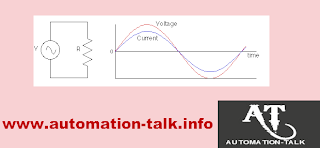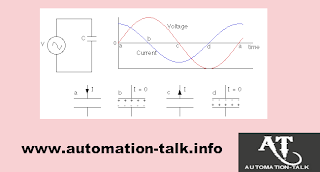## Resistance & Capacitance In AC Circuits

As we all know that AC stands for "Alternating Current". As compared to DC where current always flows in one direction, in AC instead of constant voltage, the voltage oscillates in sinusoidal wave varying with time. Voltage = V"Sinwt where w is angular frequency.

The Angular frequency is related with frequency as:-

w=2*3.14*F

The V" in the formula represents the maximum voltage and called as RMS (root mean Square) value.

RMS Value = 0.707 * Peak Value

## How to Find Resistance in AC Circuits:-

As Resistors are linear Devices so the formula V = IR also applies here.
Therefore , I = V/R = V"sin(wt)/R = I"sin(wt)In the AC Circuits consisting only of Resistors, the Phase of Current and Voltage are same. So, it means that when the Voltage is at peak then at the same time current value is also at peak.

### How to Find Capacitance in AC Circuits:-

The capacitor is used in circuits for storing charge. Consider a circuit consisting only capacitor and an AC power source. The current leads the voltage by 90 deg in Capacitive AC Circuits. It means Current reaches its peak value 90 deg before voltage. But why ?? To understand this we need to review some basic concepts.

See the Below Diagram.As we know Q= CV and I = dQ/dT

Now see the above diagram carefully and we will examine One cycle to see why there is 90 Deg phase difference between voltage and Current.

Recommended Article: Free PLC Softwares for Ladder Logic Programming

At Point "a" in above diagram, voltage is 0 so capacitor is uncharged. Initially, the Voltage increases quickly and the voltage across the capacitor plates is equal to power source voltage. So current builds up and the charge is deposited on capacitor plates. When the voltage is nearer to its peak value, it changes slowly and thus less current flows.

When it reaches point "b", the capacitor is fully charged and current is momentarily zero.

After Voltage reaches its peak, it starts decreasing so the capacitor should discharge now and current reverses in direction. See at point C, where the voltage passes through 0, changing rapidly so current has to be negative and large to cope up.

Between points c and d, the charge again builds up on capacitor plates but this time of opposite polarity as was earlier. As the voltage is negative and when it reaches its peak current drops to zero at point d.

After point d, the voltage again moves towards 0 and capacitor should discharge again. When the voltage reaches zero it completes one complete cycle.

A capacitor in AC Circuit has got some resistance called as Capacitive Reactance and it depends on the frequency of the AC voltage.
Xc = 1/wc = 1/2*3.14*Fc

To get the voltage across the capacitor we can use V = I * Xc
(Imp Note:- V and I are the RMS Values)

The larger is the capacitance of capacitor more charge has to flow to build up the required voltage on the capacitor plates and thus more current. The more is the frequency, more rapid is the voltage change and thus larger the current. Thus the current increases with the capacitance and frequency.

You can also subscribe to get all latest updates by e-mail. Subscribe to Automation-Talk by Email.

#### 1 comment:

1.This is my first time i visit here. I found so many useful stuff in your website especially its discussion; from the lot of comments on your articles! I guess I’m not the only one receiving the many satisfaction right here! Keep up a good job